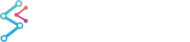﻿ Axis Layout - Vertically Stacked Axis | JavaScript Chart DocumentationSciChart.js JavaScript 2D Charts API > Axis APIs > Axis Layout - Vertically Stacked Axis
Axis Layout - Vertically Stacked Axis

Stacked axes allow you to have multiple series plotted against different axes on the same sciChartSurface, where only a portion of each axis is visible, but the series themselves can overlap.  For example:To do this you need to replace the appropriate layoutStrategy property on the layoutManager with the stacked version. SciChart provides the following Outer Axes Layout Strategies:

• LeftAlignedOuterVerticallyStackedAxisLayoutStrategy
• RightAlignedOuterVerticallyStackedAxisLayoutStrategy
• TopAlignedOuterHorizontallyStackedAxisLayoutStrategy
• BottomAlignedOuterHorizontallyStackedAxisLayoutStrategy

In the case above we use

sciChartSurface.layoutManager.leftOuterAxesLayoutStrategy = new LeftAlignedOuterVerticallyStackedAxisLayoutStrategy();

Make sure to assign Layout Strategy to an appropriate property on the Layout Manager accordingly to Axis Alignment.

Below is the full code for this example.  Note the use of xAxisId and yAxisId properties on the rendeabelSeries to tell them which axes to use.

Inner Axis Example

Copy Code
import { XyDataSeries } from "scichart/Charting/Model/XyDataSeries";
import { SciChartSurface } from "scichart";
import { EAxisAlignment } from "scichart/types/AxisAlignment";
import { NumericAxis } from "scichart/Charting/Visuals/Axis/NumericAxis";
import { LeftAlignedOuterVerticallyStackedAxisLayoutStrategy } from "scichart/Charting/LayoutManager/LeftAlignedOuterVerticallyStackedAxisLayoutStrategy";
import { FastLineRenderableSeries } from "scichart/Charting/Visuals/RenderableSeries/FastLineRenderableSeries";
import { NumberRange } from "scichart/Core/NumberRange";
/...
const { sciChartSurface, wasmContext } = await SciChartSurface.create(divElementId);
const ID_X_AXIS_1 = "xAxis1";
const ID_Y_AXIS_1 = "yAxis1";
const ID_Y_AXIS_2 = "yAxis2";
const ID_Y_AXIS_3 = "yAxis3";
// create x axis
const xAxis1 = new NumericAxis(wasmContext, { id: ID_X_AXIS_1, axisTitle: ID_X_AXIS_1, axisAlignment: EAxisAlignment.Top });
// Create y axes
const yAxis1 = new NumericAxis(wasmContext, { id: ID_Y_AXIS_1, axisTitle: ID_Y_AXIS_1, axisAlignment: EAxisAlignment.Left, visibleRange: new NumberRange(-2, 3) });
const yAxis2 = new NumericAxis(wasmContext, { id: ID_Y_AXIS_2, axisTitle: ID_Y_AXIS_2, axisAlignment: EAxisAlignment.Left, visibleRange: new NumberRange(-2, 2) });
const yAxis3 = new NumericAxis(wasmContext, { id: ID_Y_AXIS_3, axisTitle: ID_Y_AXIS_3, axisAlignment: EAxisAlignment.Left, visibleRange: new NumberRange(-3, 2) });
// Add all axes to surface
// This will cause axes with axisAlignment equal to EAxisAlignment.Left to be stacked up vertically
sciChartSurface.layoutManager.leftOuterAxesLayoutStrategy = new LeftAlignedOuterVerticallyStackedAxisLayoutStrategy();
yAxis2.axisBorder.borderTop = 20;
yAxis2.axisBorder.borderBottom = 20;
// Create renderable series
const lineSeries1 = new FastLineRenderableSeries(wasmContext, {
yAxisId: ID_Y_AXIS_1,
xAxisId: ID_X_AXIS_1,
stroke: "yellow",
});
const lineSeries2 = new FastLineRenderableSeries(wasmContext, {
yAxisId: ID_Y_AXIS_2,
xAxisId: ID_X_AXIS_1,
stroke: "red",
});
const lineSeries3 = new FastLineRenderableSeries(wasmContext, {
yAxisId: ID_Y_AXIS_3,
xAxisId: ID_X_AXIS_1,
stroke: "blue",
});
// Set data
lineSeries1.dataSeries = getSinewave(wasmContext, 0, 3, 1, 0, 1000, 10);
lineSeries2.dataSeries = getSinewave(wasmContext, 0, 3, 2, 0, 1000, 10);
lineSeries3.dataSeries = getSinewave(wasmContext, 0, 3, 3, 0, 1000, 10);
// Add renderable series to the surface

This is the getSinewave function:

Get sinewave data

Copy Code
function getSinewave(
wasmContext,
amplitude,
phase,
dampingFactor,
pointCount,
freq,
) {
const dataSeries = new XyDataSeries(wasmContext);
for (let i = 0; i < pad; i++) {
const time = 10 * i / pointCount;
dataSeries.append(time, 0);
}
for (let i = pad, j = 0; i < pointCount; i++, j++) {
const time = 10 * i / pointCount;
const wn = 2 * Math.PI / (pointCount / freq);
const d = amplitude * Math.sin(j * wn + phase);
dataSeries.append(time, d);
amplitude *= (1.0 - dampingFactor);
}
return dataSeries;
}